# EECS101001 Homework 3 Solved

35.00 \$ 17.50 \$

Category:
Click Category Button to View Your Next Assignment | Homework

You'll get a download link with a: . ` zip` solution files instantly, after Payment

## Description

Rate this product
1.  Simplify the following Boolean functions or expression, using three-variable maps:
•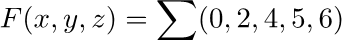•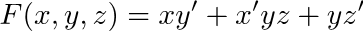1.  Simplify the following Boolean functions by first finding the essential prime implicants (Please indicate the essential prime implicants and prime implicants):
•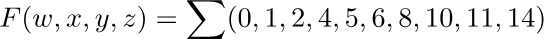•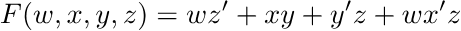1.  Simplify the following expressions in (a) sum of products and (b) product of sums: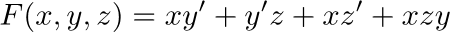1.  Simplify the following Boolean function F, together with the don’t-care conditions d, and then express the simplified function in sum of products:
•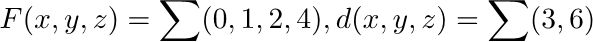•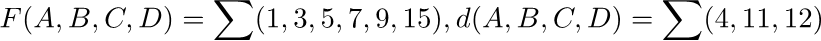1.  Simplify the following expression, and implement it with two-level NAND gates:1. Simplify the following expression, and implement it with two-level NOR gates:1.  Simplify the following Boolean function F, using the two-level forms (a) AND-OR-Inverter, (b) OR-AND-Inverter logic diagrams